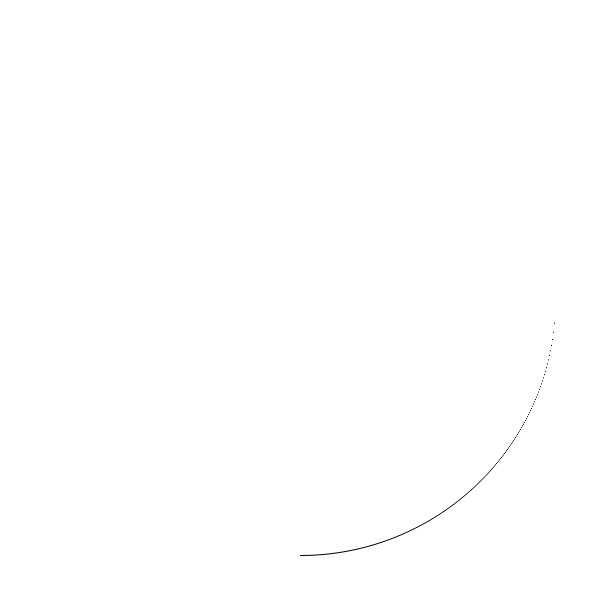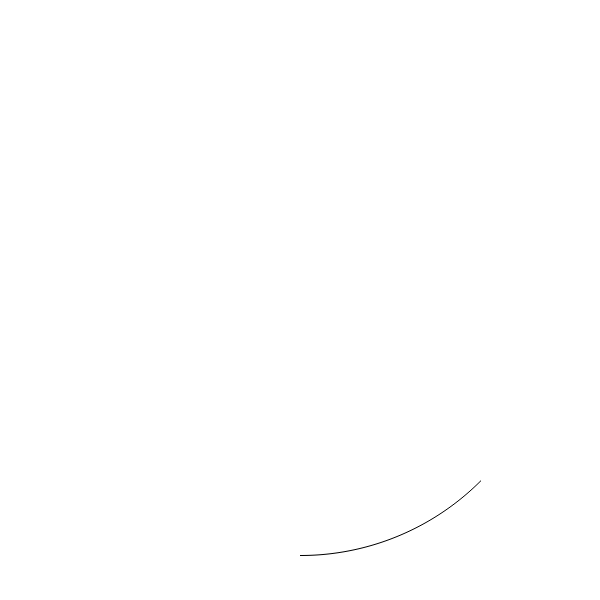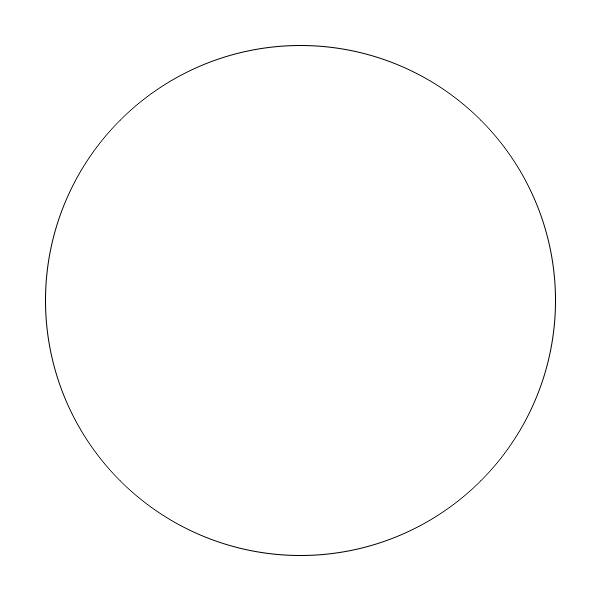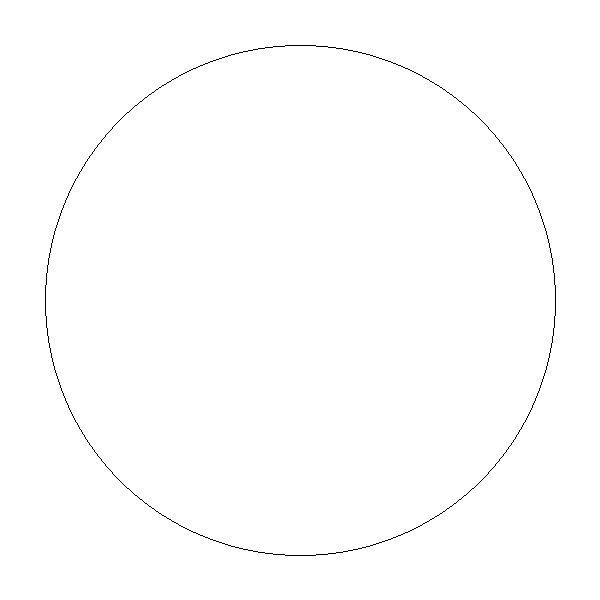## [Math] Drawing a circle, point-by-point, without floating point support

Now we need to add a circle to our clock.

Circle is defined by Pythagorean theorem: $x^2 + y^2 = r^2$, where $x$ and $y$ -- coordinates on plain and $r$ is radius.

### Naive algorithm

So let's draw it! Enumerate all points in 0..radius range and find "height" for each point. Height will be $\sqrt{r^2 - y^2}$ We will draw only a quadrant, i.e., $\frac{1}{4}$ of the circle:

<!doctype html>
<html>
<style>
#my-canvas { border: 1px solid gray; }
</style>
<body>
<canvas id="my-canvas" width="600" height="600"></canvas>
<script>

var canvas = document.querySelector('#my-canvas');
var context = canvas.getContext('2d');
var half_width = canvas.width/2;
var half_height = canvas.height/2;
// clock is slightly smaller than canvas:

function putPixel(x, y)
{
context.fillRect(x+half_width, y+half_height, 1, 1);
};

function circle()
{
// x^2 + y^2 = radius^2
// x^2 = radius^2 - y^2
{
putPixel(x, y);
}
};

circle();

</script>
</body>
</html>Ouch! This arc looks correct, but the points at right-bottom are "dispersed".

The common way is to draw only octant ($\frac{1}{8}$) of a circle and then "copy" the octant 7 times more. But to draw a 45° angle (or $\frac{\Pi}{4}$) you have to use some trigonometry.

      function circle()
{
// Pi/4 = octant. find a point on x where octant is ended:
var octant_limit = clock_radius * Math.cos(Math.PI/4);

for (x=0; x<octant_limit; x++)
{
putPixel(x, y);
}
};


Looks nice:Now copy it 7 more times:

          for (x=0; x<octant_limit; x++)
{
putPixel(x, y);
// copy our octant 7 times:
putPixel(x, -y);
putPixel(-x, y);
putPixel(-x, -y);

putPixel(y, x);
putPixel(y, -x);
putPixel(-y, x);
putPixel(-y, -x);
}### Midpoint circle algorithm

Naive algorithm is correct, but it requires at least one "heavy" operation -- square root.

Here I will rework my algorithm a bit:

      function circle(x0, y0, radius)
{
// starting point:
var y = 0;

while (x >= y)
{
// main octant:
putPixel(x0 + x, y0 + y);
// copy octant 7 times:
putPixel(x0 + y, y0 + x);
putPixel(x0 - y, y0 + x);
putPixel(x0 - x, y0 + y);
putPixel(x0 - x, y0 - y);
putPixel(x0 - y, y0 - x);
putPixel(x0 + y, y0 - x);
putPixel(x0 + x, y0 - y);

y=y+1;
var new_radius=Math.sqrt(x**2 + y**2).toFixed(); // also convert to int
// we check here if the 'new radius' becomes too big
// if so, decrease $x$
// we do this to maintain the equation x^2 + y^2 = r^2 (for integer arithmetic)
x=x-1;
}
};We "maintain" the equation here. If radius becomes too big, we get "stray" point back, so that it will always lay on circle's edge.

And this algorithm has also square root operation.

Please note -- we recalculate "new_radius" at each iteration. Can we "update" that value rather than scratching from start? "Adjust" it?

Yes, if we know a bits of calculus. I described it earlier. Instead of recalculating $x^2$ each time, we can add derivative to some temporary variable: $2x + 1$.

The function will be different now:

      function circle(x0, y0, radius)
{
// starting point:
var y = 0;

while (x >= y)
{
// main octant:
putPixel(x0 + x, y0 + y);
// copy octant 7 times:
putPixel(x0 + y, y0 + x);
putPixel(x0 - y, y0 + x);
putPixel(x0 - x, y0 + y);
putPixel(x0 - x, y0 - y);
putPixel(x0 - y, y0 - x);
putPixel(x0 + y, y0 - x);
putPixel(x0 + x, y0 - y);

// increase $y$ and update $current_radius$
y += 1;

// if $current_radius$ is bigger than $radius$, decrease $x$ and update $current_radius$ again
// we do this to maintain the equation x^2 + y^2 = r^2 (for integer arithmetic)
{
x -= 1;
}
}
};


Please note: we don't maintain current radius anymore. We maintain it in "squared" form. current_radius_squared is always equals to the $x^2 + y^2$ expression.

So if in the expression $x^2 + y^2$, $x$ is increased by 1, just add $2x + 1$ to it. If decreased by 1, subtract. No need to calculate power(s).

Also, since we precomputed $r^2$ value at start, we can use at as "reference" value.

Isn't it cool to apply some calculus to a real-life problem?

The third version is merely an optimization. Here we don't maintain current radius, we maintain only error or difference between radius-we-have and radius-must-be.

This version is highly popular and you'll find it when googling for the midpoint circle algorithm.

      function circle(x0, y0, radius)
{
// starting point:
var y = 0;

var err = 0;

while (x >= y)
{
// main octant:
putPixel(x0 + x, y0 + y);
// copy octant 7 times:
putPixel(x0 + y, y0 + x);
putPixel(x0 - y, y0 + x);
putPixel(x0 - x, y0 + y);
putPixel(x0 - x, y0 - y);
putPixel(x0 - y, y0 - x);
putPixel(x0 + y, y0 - x);
putPixel(x0 + x, y0 - y);

// increase $y$ and update $err$
y += 1;
err += 2*y + 1;

// if $err$ is bigger than zero, decrease $x$ and update $err$ again
// we do this to maintain the equation x^2 + y^2 - r^2 = 0 (for integer arithmetic)
if (err > 0)
{
x -= 1;
err -= 2*x + 1;
}
}
};


The final optimized version is cool. It requires only additions, subtractions and bit shifts: $2x$ is the same as x<<1, of course. It also requires only integer arithmetic.

Summary: many explanations of midpoint algorithm use the final, optimized version. But I added several unoptimized steps.

There are couple of blog posts I read to better understanding. Mukund Sivaraman, Neeraj Mishra.

As seen at lobste.rs, HN.Yes, I know about these lousy Disqus ads. Please use adblocker. I would consider to subscribe to 'pro' version of Disqus if the signal/noise ratio in comments would be good enough.